## Saturday, October 15, 2022

### Fitting an Mk model to discrete character data when the states at some (or all) internal nodes are known

Recently, a phytools user asked the following question:

“We have a situation where we happen to (think we) know the ancestral state - is there a way in phytools to set/define it or define the ancestral frequencies of characters? (To then estimate Mk parameters and/or do model testing.) Sorry if this is a stupid question.”

The answer to this (non-stupid) question is, of course, yes!

How we do it, though, will depend on whether we're talking about the global root of the tree, or about any other arbitrary internal node.

Much as I hate to, to best illustrate this I'll use a simulated discrete character – because that is (effectively) the only way that I genuinely know the true ancestral states!

I'll use a real, empirical tree, however: in this case, a phylogeny of 258 species of liolaemid lizards (and kin) from a paper by Damien Esquerré et al. (2019).

``````library(phytools)
lizard.tree
``````
``````##
## Phylogenetic tree with 258 tips and 257 internal nodes.
##
## Tip labels:
##   Ctenoblepharys_adspersa, Liolaemus_abaucan, Liolaemus_koslowskyi, Liolaemus_albiceps, Liolaemus_irregularis, Liolaemus_ornatus, ...
##
## Rooted; includes branch lengths.
``````

Now, I'll simulate a character with three states (a, b, and c) on the phylogeny.

I'll do this in such a way (using `phytools::sim.history` instead of `phytools::sim.Mk`) that it'll be easy for me to pull out both the observed tip states and all the states at the internal nodes of the phylogeny.

I can start by setting my Q matrix. This is the parameter of the Mk model that we'll be trying to estimate. Here I use an arbitrary value of Q – but one in which the rate of transition from one state to the others tends to be asymmetrical.

``````Q<-matrix(c(
-0.12,0.1,0.02,
0.05,-0.10,0.05,
0.00,0.15,-0.15),3,3,
dimnames=list(letters[1:3],letters[1:3]),
byrow=TRUE)
plot(as.Qmatrix(Q),color=TRUE,width=TRUE)
title(main="simulated Q (transition) matrix",font.main=3,
line=-1)
``````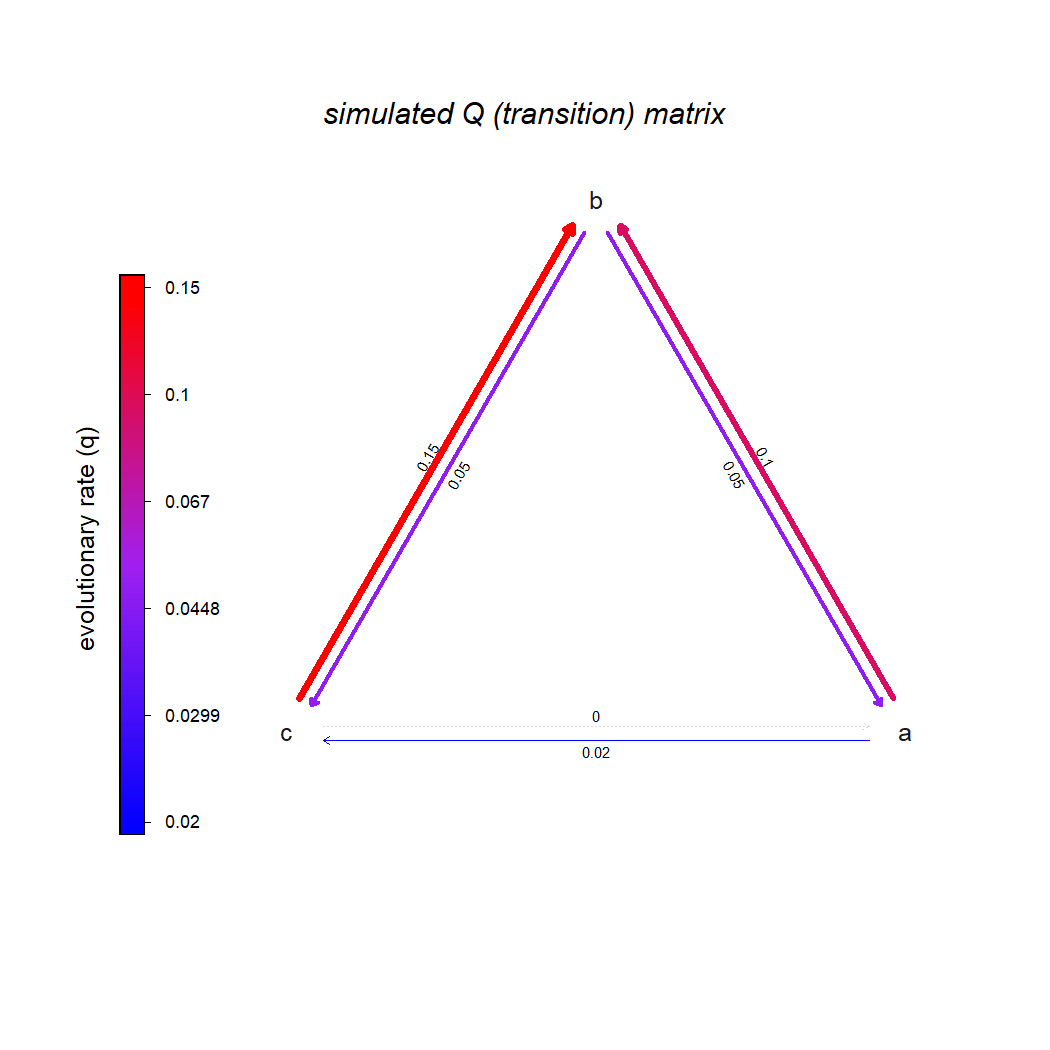Next, let's simulate our true history, under this model.

``````tt<-sim.history(lizard.tree,Q,direction="row_to_column")
``````
``````## Note - the rate of substitution from i->j should be given by Q[i,j].
## Done simulation(s).
``````
``````cols<-setNames(RColorBrewer::brewer.pal(3,"Dark2"),
letters[1:3])
plot(tt,cols,ftype="off",mar=c(0.1,1.1,4.1,1.1))
legend("topleft",letters[1:3],col=cols,
pch=15,cex=0.8,pt.cex=1.2,bty="n")
title(main="true character history",font.main=3)
``````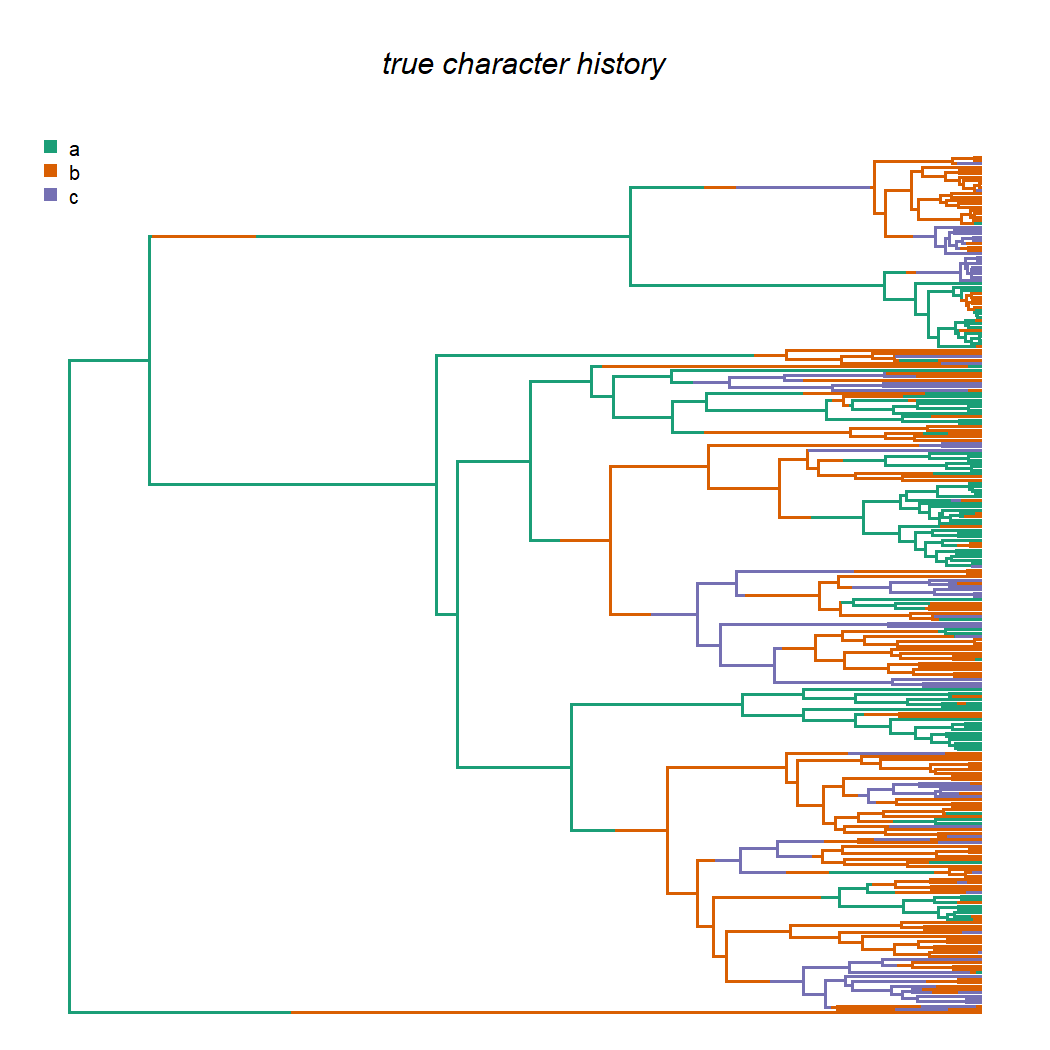Then, let's pull out the tip (species) states and the internal node states in separate vectors. To do this, I'll use the function `phytools::getStates` as follows.

``````x<-as.factor(getStates(tt,"tips"))
``````
``````## Ctenoblepharys_adspersa       Liolaemus_abaucan    Liolaemus_koslowskyi      Liolaemus_albiceps   Liolaemus_irregularis
##                       b                       b                       b                       c                       c
##       Liolaemus_ornatus
##                       c
## Levels: a b c
``````
``````nn<-as.factor(getStates(tt,"nodes"))
``````
``````## 259 260 261 262 263 264
##   a   a   a   a   a   b
## Levels: a b c
``````

Here we can see all of our tip and node states from the simulation above.

``````plotTree(lizard.tree,ftype="off",lwd=1,type="fan")
par(fg="transparent")
tiplabels(pie=to.matrix(x[lizard.tree\$tip.label],levels(x)),piecol=cols,
cex=0.3)
nodelabels(pie=to.matrix(nn,levels(nn)),piecol=cols,cex=0.3)
``````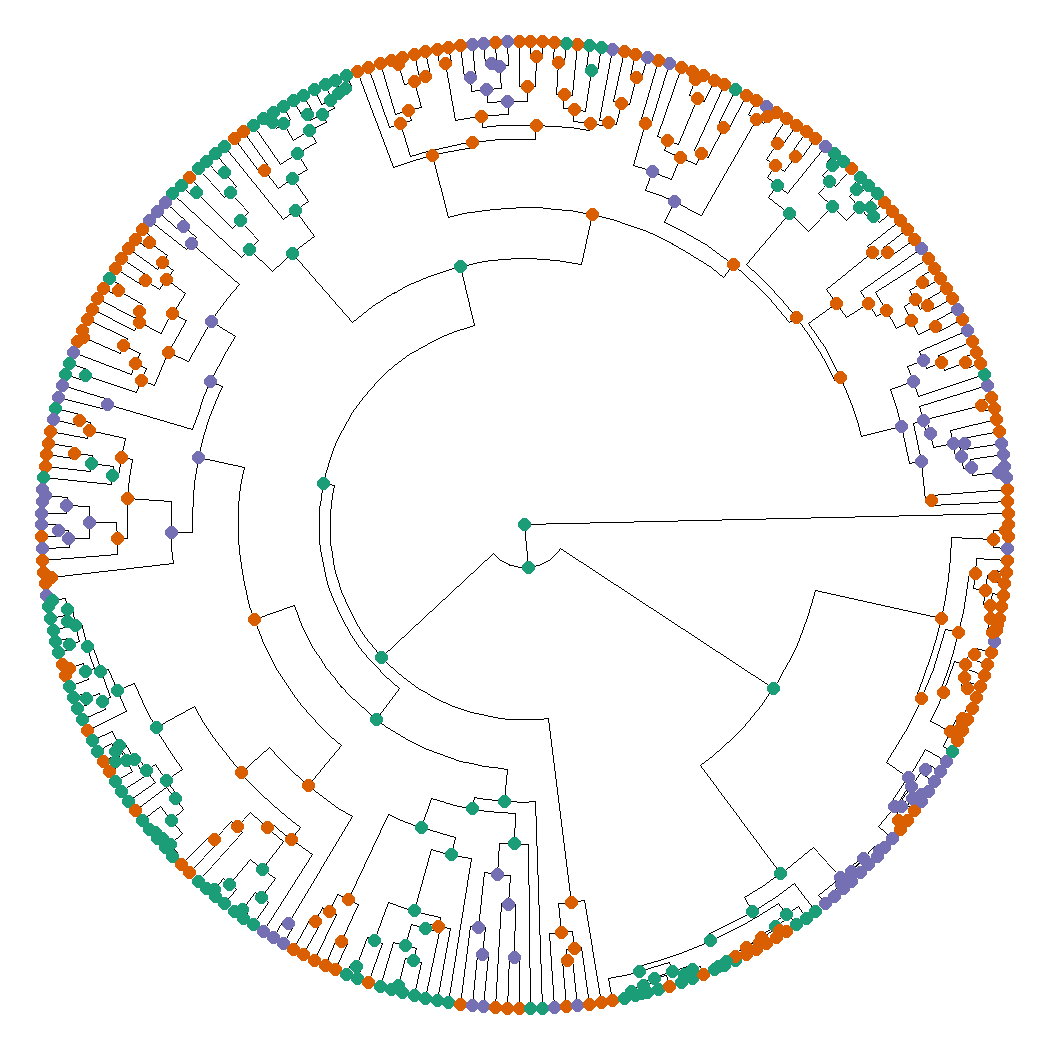``````par(fg="black")
``````

So far, so good.

To start, let's imagine we don't know anything about the internal nodes of the tree.

In this case, we would just fit a standard (extended) Mk model, which I'll do here using `fitMk.parallel` (merely because it provides slightly more reliable optimization for larger trees).

After I fit the model, I'll also graph it using `plot.Qmatrix` in the phytools package.

``````fitARD.no_nodes<-fitMk.parallel(lizard.tree,x,model="ARD",pi="fitzjohn")
fitARD.no_nodes
``````
``````## Object of class "fitMk".
##
## Fitted (or set) value of Q:
##           a         b         c
## a -0.093154  0.084267  0.008887
## b  0.042270 -0.107639  0.065369
## c  0.000000  0.170142 -0.170142
##
## Fitted (or set) value of pi:
##        a        b        c
## 0.347079 0.329664 0.323257
## due to treating the root prior as (a) nuisance.
##
## Log-likelihood: -200.097673
##
## Optimization method used was "optimParallel"
``````
``````plot(fitARD.no_nodes,width=TRUE,color=TRUE,tol=1e-3,offset=0.025)
title(main="estimated Q matrix with NO internal node information",
font.main=3,line=-1)
``````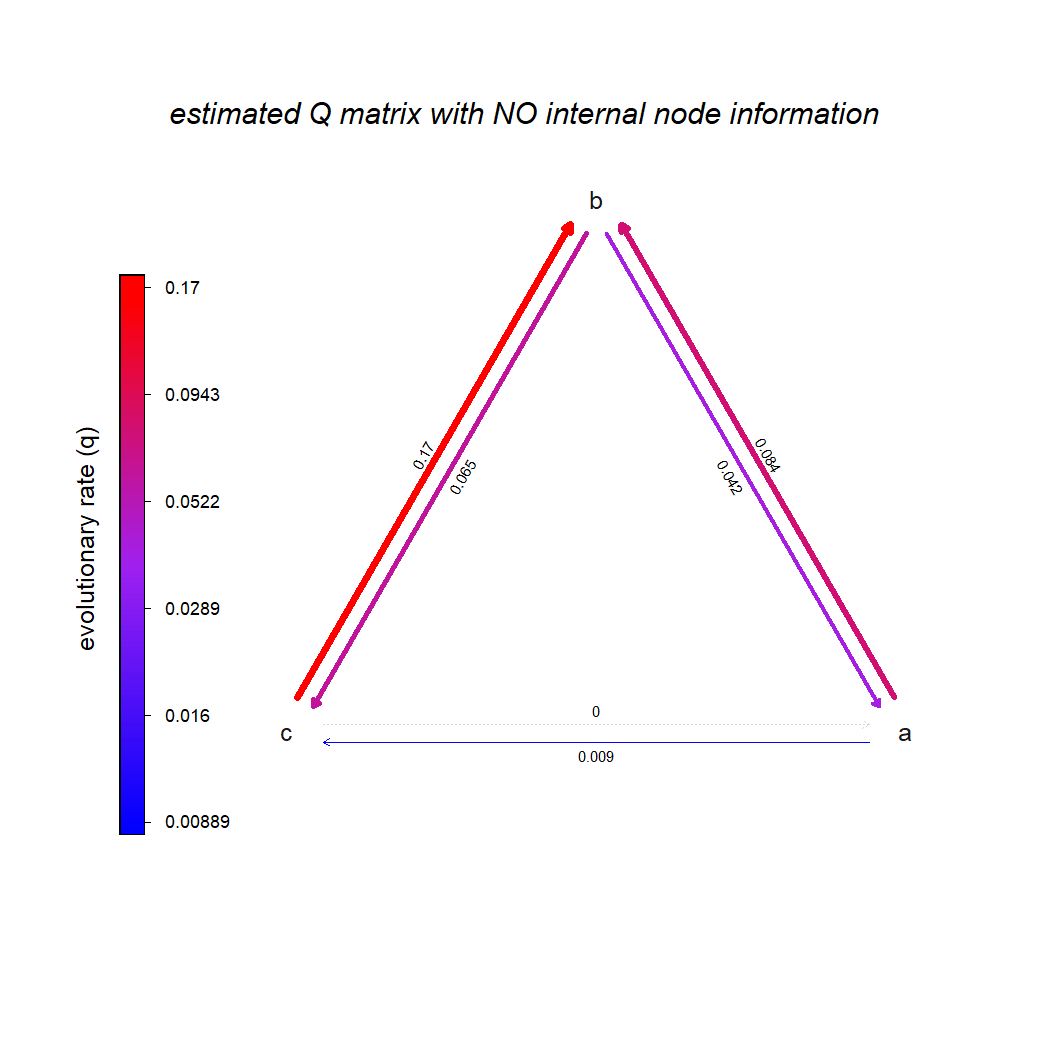Next, let's deal with the very simple case of knowing just the global root of the tree.

Here, we'll just fix the root to its known value using the argument `pi` (the prior probabilities at the root), which we have to supply in the form of a vector.

In this instance I'll pull my vector out of the known states for all internal nodes using `phytools::to.matrix` – but ordinarily we would just supply a custom vector of our own design.

``````pi<-to.matrix(nn,levels(nn))[1,]
pi ## this is what pi should look like
``````
``````## a b c
## 1 0 0
``````
``````fitARD.root_node<-fitMk.parallel(lizard.tree,x,model="ARD",
pi=pi)
fitARD.root_node
``````
``````## Object of class "fitMk".
##
## Fitted (or set) value of Q:
##           a         b         c
## a -0.093002  0.084125  0.008877
## b  0.041585 -0.107029  0.065444
## c  0.000000  0.169250 -0.169250
##
## Fitted (or set) value of pi:
## a b c
## 1 0 0
## due to treating the root prior as (a) given.
##
## Log-likelihood: -200.056343
##
## Optimization method used was "optimParallel"
``````
``````plot(fitARD.root_node,width=TRUE,color=TRUE,tol=1e-3,
offset=0.025)
title(main="estimated Q matrix with KNOWN global root",
font.main=3,line=-1)
``````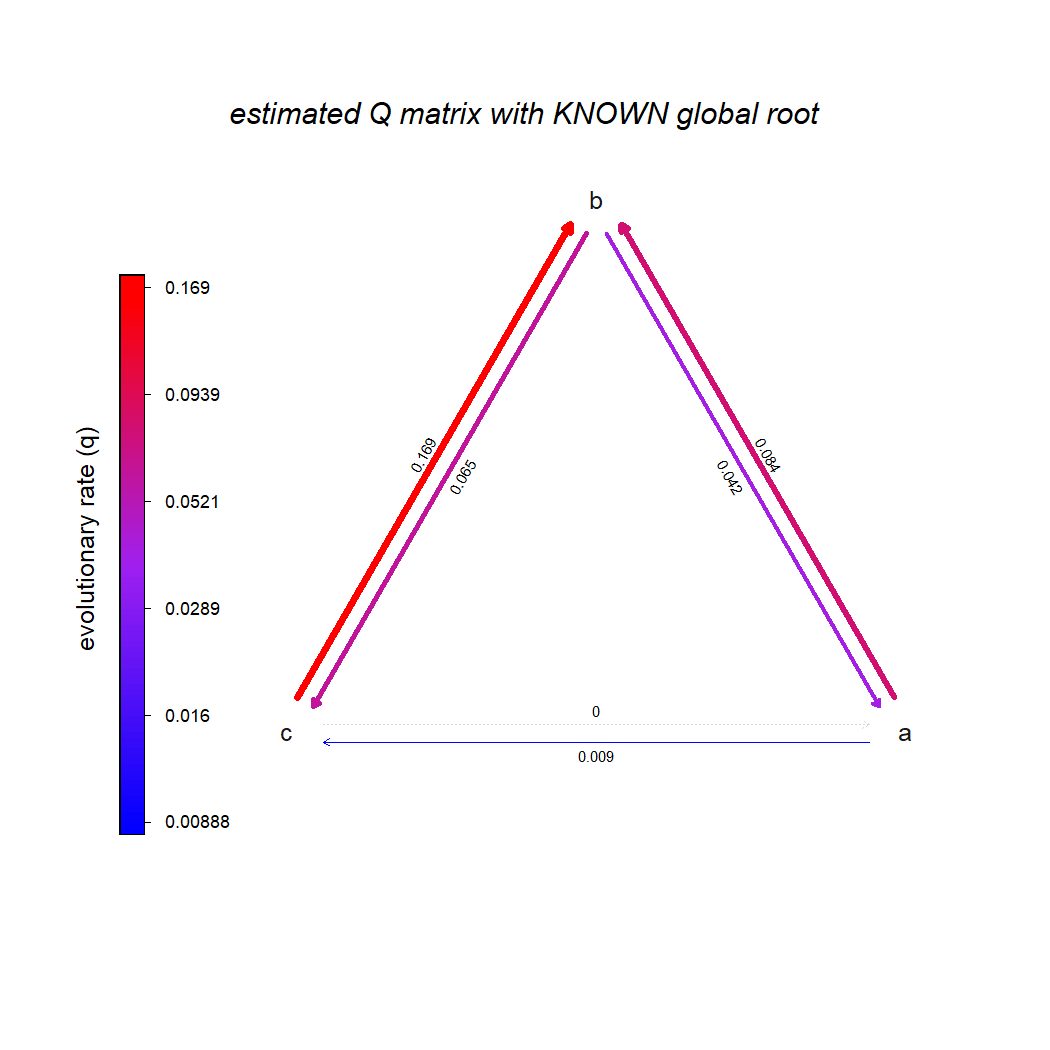Next, let's imagine the very optimistic scenario in which we know (that is to say, unambigiously) the states at 30 of the 257 internal nodes of our phylogeny.

In this case, unfortunately, `fitMk` does not provide us with any easy way to specify these values.

What we can do, however, is just add 30 zero-length tips at all the nodes for which we have knowledge, and then supply these values as additional tip states.

For my example, I'll simply choose 30 nodes at random from all 257 in my tree, as follows.

``````Nodes<-nn[mn<-sort(sample(1:lizard.tree\$Nnode,30))]
Nodes ## these are our imagined known states
``````
``````## 260 274 281 290 297 299 303 313 319 321 329 330 335 359 360 368 401 402 413 416 421 422 429 451 464 493 503 505 507 513
##   a   c   b   b   a   a   b   b   b   b   b   b   b   a   b   b   a   a   a   a   a   a   a   c   a   b   b   b   b   b
## Levels: a b c
``````

In any practical empirical scenario, these would be the node values and node indices of our known internal states in a vector.

Now, I'll add my terminal zero-length edges & visualize all the data that we have (our tips & known internal nodes, but drawn as tips) on the corresponding tree.

``````temp<-lizard.tree
for(i in 1:length(Nodes)){
M<-matchNodes(lizard.tree,temp)
node<-as.numeric(names(Nodes)[i])
temp<-bind.tip(temp,node,edge.length=0,
where=M[which(M[,1]==node),2])
cat(".")
flush.console()
if(i==length(Nodes)) cat("\n")
}
``````
``````## Adding zero-length tips:
## ..............................
``````
``````plotTree(temp,ftype="off",lwd=1,type="fan")
par(fg="transparent")
tiplabels(pie=to.matrix(c(x,Nodes)[temp\$tip.label],levels(x)),piecol=cols,
cex=0.3)
par(fg="black")
legend("topleft",letters[1:3],col=cols,
pch=15,cex=0.8,pt.cex=1.2,bty="n")
``````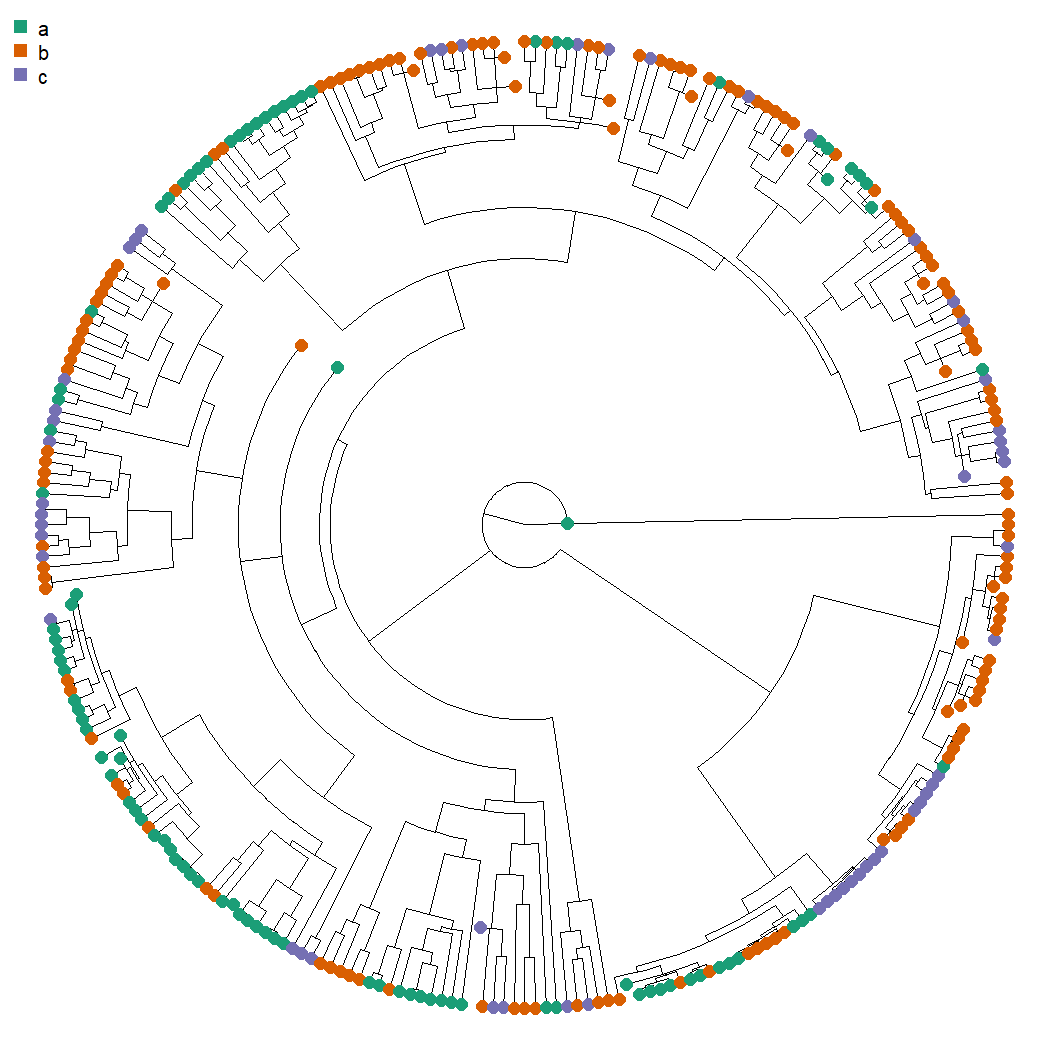Lastly, I'll fit our model to this tree and data!

``````fitARD.many_nodes<-fitMk.parallel(temp,c(x,Nodes),model="ARD",
pi="fitzjohn",ncores=8)
fitARD.many_nodes
``````
``````## Object of class "fitMk".
##
## Fitted (or set) value of Q:
##           a         b         c
## a -0.090087  0.082737  0.007350
## b  0.036875 -0.098168  0.061293
## c  0.000000  0.146881 -0.146881
##
## Fitted (or set) value of pi:
##        a        b        c
## 0.847970 0.120433 0.031597
## due to treating the root prior as (a) nuisance.
##
## Log-likelihood: -204.803337
##
## Optimization method used was "optimParallel"
``````
``````plot(fitARD.many_nodes,width=TRUE,color=TRUE,tol=1e-3,
offset=0.025)
title(main="estimated Q matrix with 30 KNOWN internal nodes",
font.main=3,line=-1)
``````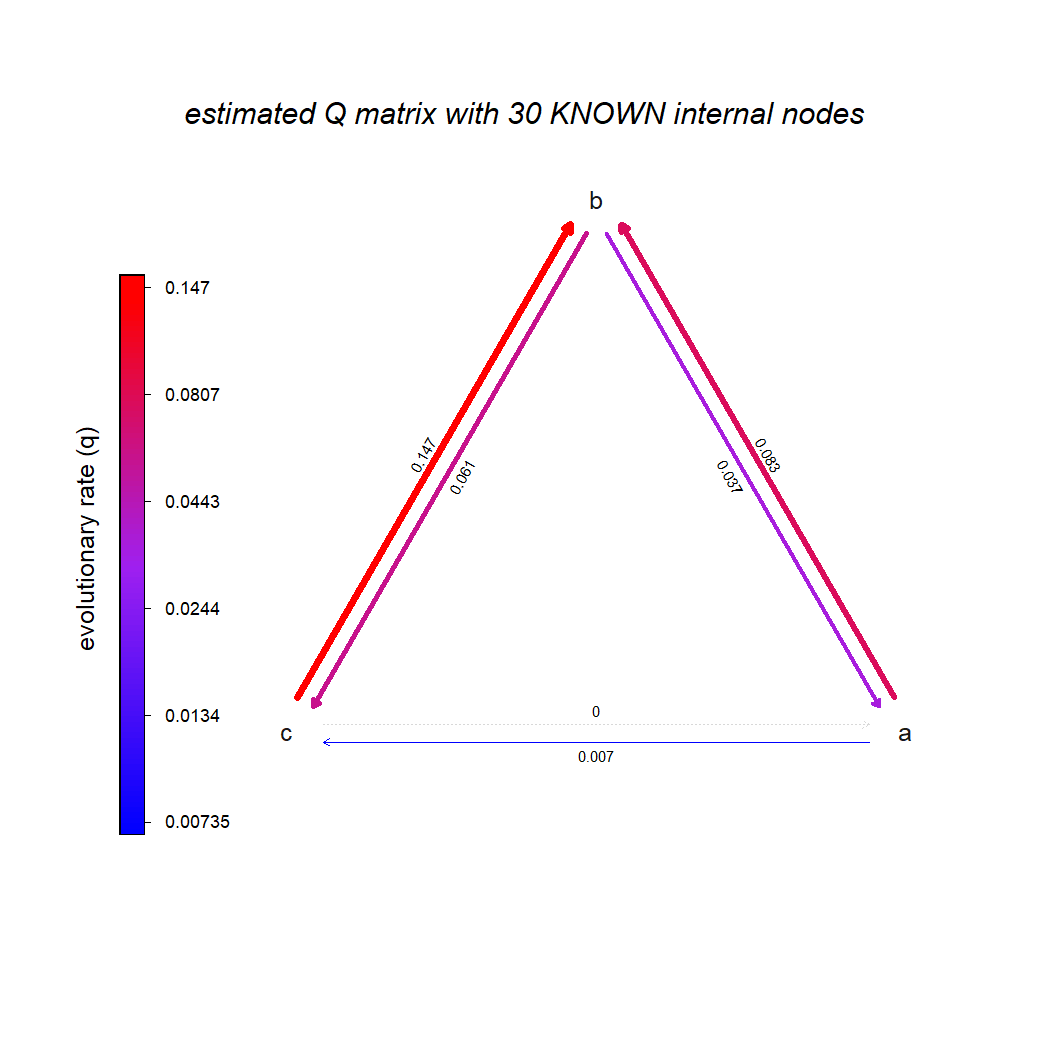Hopefully, what we're seeing is that for more & more information about internal nodes, our solution converges closer & closer to the generating Q matrix!

Lastly, just for fun, let's imagine fitting the same model – but with all internal node states.

This is what that might look like.

``````Nodes<-nn
temp<-lizard.tree
for(i in 1:length(Nodes)){
M<-matchNodes(lizard.tree,temp)
node<-as.numeric(names(Nodes)[i])
temp<-bind.tip(temp,node,edge.length=0,
where=M[which(M[,1]==node),2])
cat(".")
flush.console()
if(i==length(Nodes)||i%%30==0) cat("\n")
}
``````
``````## Adding zero-length tips:
## ..............................
## ..............................
## ..............................
## ..............................
## ..............................
## ..............................
## ..............................
## ..............................
## .................
``````
``````fitARD.all_nodes<-fitMk.parallel(temp,c(x,Nodes),model="ARD",
pi="fitzjohn",ncores=8)
fitARD.all_nodes
``````
``````## Object of class "fitMk".
##
## Fitted (or set) value of Q:
##           a         b         c
## a -0.089987  0.081160  0.008827
## b  0.041896 -0.096062  0.054166
## c  0.000000  0.136344 -0.136344
##
## Fitted (or set) value of pi:
## a b c
## 1 0 0
## due to treating the root prior as (a) nuisance.
##
## Log-likelihood: -255.041214
##
## Optimization method used was "optimParallel"
``````
``````plot(fitARD.all_nodes,width=TRUE,color=TRUE,tol=1e-3,
offset=0.025)
title(main="estimated Q matrix with ALL internal nodes known",
font.main=3,line=-1)
``````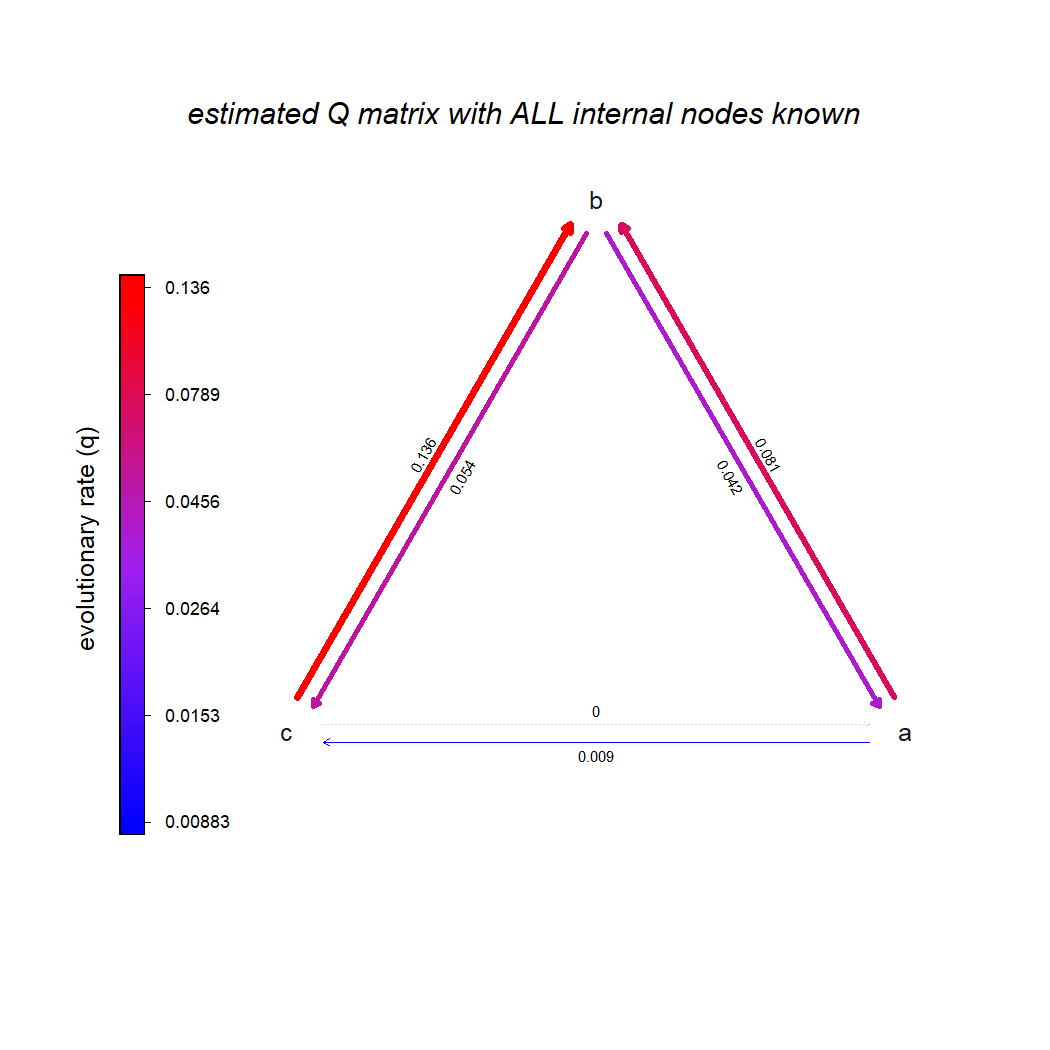One small observation: the log-likelihoods of these different fitted models are not comparable. That's simply because they've been obtained using different data.

That's it!